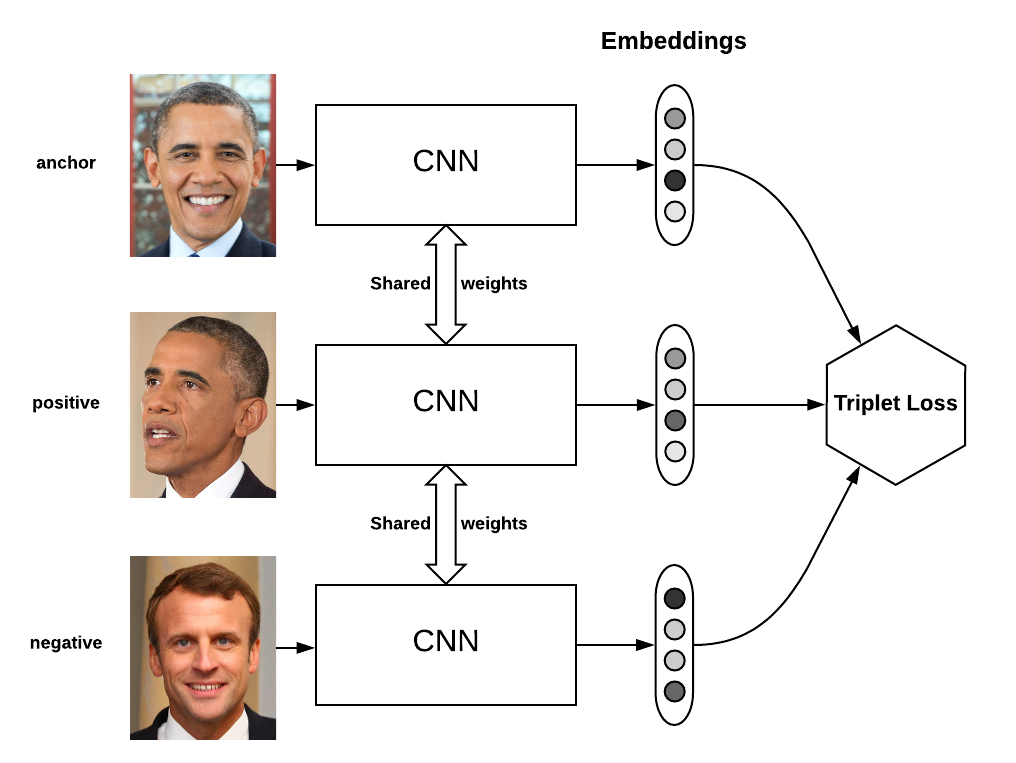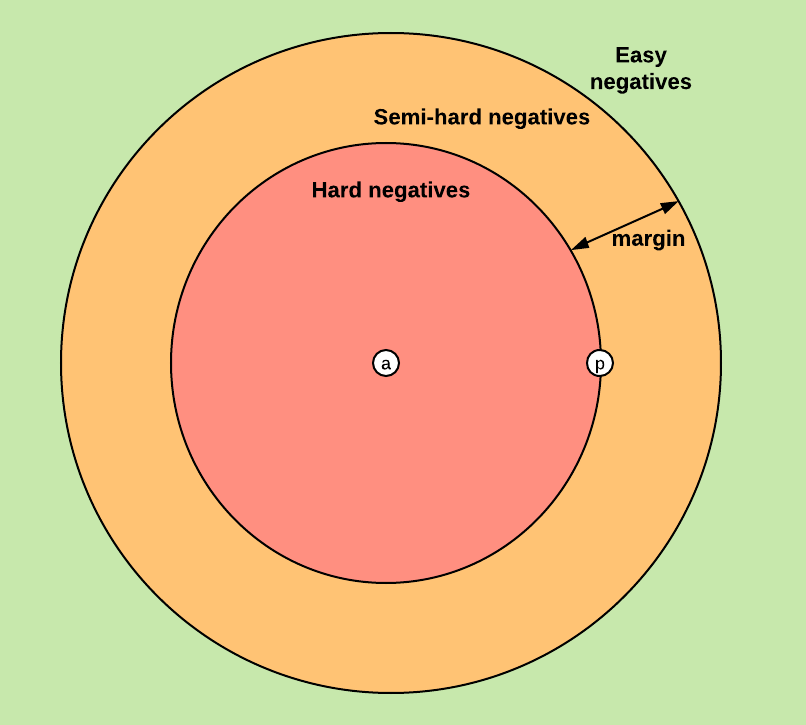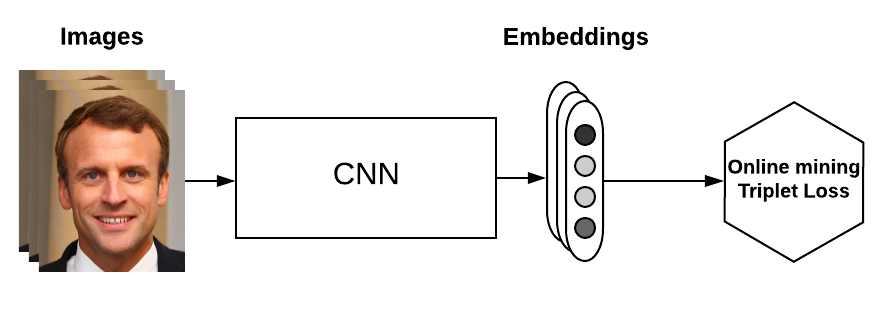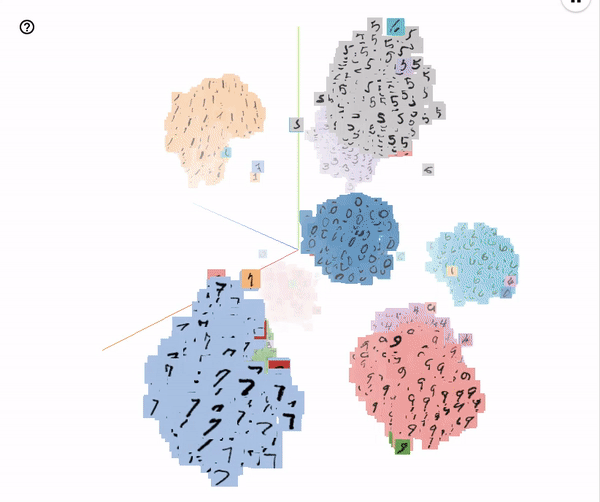In face recognition, triplet loss is used to learn good embeddings (or “encodings”) of faces. If you are not familiar with triplet loss, you should first learn about it by watching this coursera video from Andrew Ng’s deep learning specialization.

Triplet loss is known to be difficult to implement, especially if you add the constraints of building a computational graph in TensorFlow.

In this post, I will define the triplet loss and the different strategies to sample triplets. I will then explain how to correctly implement triplet loss with online triplet mining in TensorFlow.

About two years ago, I was working on face recognition during my internship at Reminiz and I answered a question on stackoverflow about implementing triplet loss in TensorFlow. I concluded by saying:

Clearly, implementing triplet loss in Tensorflow is hard, and there are ways to make it more efficient than sampling in python but explaining them would require a whole blog post !

Two years later, here we go.

All the code can be found on this github repository.

## Triplet loss and triplet mining

### Why not just use softmax?

The triplet loss for face recognition has been introduced by the paper FaceNet: A Unified Embedding for Face Recognition and Clustering from Google. They describe a new approach to train face embeddings using online triplet mining, which will be discussed in the next section.

Usually in supervised learning we have a fixed number of classes and train the network using the softmax cross entropy loss. However in some cases we need to be able to have a variable number of classes. In face recognition for instance, we need to be able to compare two unknown faces and say whether they are from the same person or not.

Triplet loss in this case is a way to learn good embeddings for each face. In the embedding space, faces from the same person should be close together and form well separated clusters.

### Definition of the lossTriplet loss on two positive faces (Obama) and one negative face (Macron)

The goal of the triplet loss is to make sure that:

• Two examples with the same label have their embeddings close together in the embedding space
• Two examples with different labels have their embeddings far away.

However, we don’t want to push the train embeddings of each label to collapse into very small clusters. The only requirement is that given two positive examples of the same class and one negative example, the negative should be farther away than the positive by some margin. This is very similar to the margin used in SVMs, and here we want the clusters of each class to be separated by the margin.

To formalise this requirement, the loss will be defined over triplets of embeddings:

• an anchor
• a positive of the same class as the anchor
• a negative of a different class

For some distance on the embedding space $d$, the loss of a triplet $(a, p, n)$ is:

We minimize this loss, which pushes $d(a, p)$ to $0$ and $d(a, n)$ to be greater than $d(a, p) + margin$. As soon as $n$ becomes an “easy negative”, the loss becomes zero.

### Triplet mining

Based on the definition of the loss, there are three categories of triplets:

• easy triplets: triplets which have a loss of $0$, because $d(a, p) + margin < d(a,n)$
• hard triplets: triplets where the negative is closer to the anchor than the positive, i.e. $d(a,n) < d(a,p)$
• semi-hard triplets: triplets where the negative is not closer to the anchor than the positive, but which still have positive loss: $d(a, p) < d(a, n) < d(a, p) + margin$

Each of these definitions depend on where the negative is, relatively to the anchor and positive. We can therefore extend these three categories to the negatives: hard negatives, semi-hard negatives or easy negatives.

The figure below shows the three corresponding regions of the embedding space for the negative.The three types of negatives, given an anchor and a positive

Choosing what kind of triplets we want to train on will greatly impact our metrics. In the original Facenet paper, they pick a random semi-hard negative for every pair of anchor and positive, and train on these triplets.

### Offline and online triplet mining

We have defined a loss on triplets of embeddings, and have seen that some triplets are more useful than others. The question now is how to sample, or “mine” these triplets.

Offline triplet mining

The first way to produce triplets is to find them offline, at the beginning of each epoch for instance. We compute all the embeddings on the training set, and then only select hard or semi-hard triplets. We can then train one epoch on these triplets.

Concretely, we would produce a list of triplets $(i, j, k)$. We would then create batches of these triplets of size $B$, which means we will have to compute $3B$ embeddings to get the $B$ triplets, compute the loss of these $B$ triplets and then backpropagate into the network.

Overall this technique is not very efficient since we need to do a full pass on the training set to generate triplets. It also requires to update the offline mined triplets regularly.

Online triplet mining

Online triplet mining was introduced in Facenet and has been well described by Brandon Amos in his blog post OpenFace 0.2.0: Higher accuracy and halved execution time.

The idea here is to compute useful triplets on the fly, for each batch of inputs. Given a batch of $B$ examples (for instance $B$ images of faces), we compute the $B$ embeddings and we then can find a maximum of $B^3$ triplets. Of course, most of these triplets are not valid (i.e. they don’t have 2 positives and 1 negative).

This technique gives you more triplets for a single batch of inputs, and doesn’t require any offline mining. It is therefore much more efficient. We will see an implementation of this in the last part.Triplet loss with online mining: triplets are computed on the fly from a batch of embeddings

### Strategies in online mining

In online mining, we have computed a batch of $B$ embeddings from a batch of $B$ inputs. Now we want to generate triplets from these $B$ embeddings.

Whenever we have three indices $i, j, k \in [1, B]$, if examples $i$ and $j$ have the same label but are distinct, and example $k$ has a different label, we say that $(i, j, k)$ is a valid triplet. What remains here is to have a good strategy to pick triplets among the valid ones on which to compute the loss.

A detailed explanation of two of these strategies can be found in section 2 of the paper In Defense of the Triplet Loss for Person Re-Identification.

They suppose that you have a batch of faces as input of size $B = PK$, composed of $P$ different persons with $K$ images each. A typical value is $K = 4$. The two strategies are:

• batch all: select all the valid triplets, and average the loss on the hard and semi-hard triplets.
• a crucial point here is to not take into account the easy triplets (those with loss $0$), as averaging on them would make the overall loss very small
• this produces a total of $PK(K-1)(PK-K)$ triplets ($PK$ anchors, $K-1$ possible positives per anchor, $PK-K$ possible negatives)
• batch hard: for each anchor, select the hardest positive (biggest distance $d(a, p)$) and the hardest negative among the batch
• this produces $PK$ triplets
• the selected triplets are the hardest among the batch

According to the paper cited above, the batch hard strategy yields the best performance:

Additionally, the selected triplets can be considered moderate triplets, since they are the hardest within a small subset of the data, which is exactly what is best for learning with the triplet loss.

However it really depends on your dataset and should be decided by comparing performance on the dev set.

## A naive implementation of triplet loss

In the stackoverflow answer, I gave a simple implementation of triplet loss for offline triplet mining:

anchor_output = ...    # shape [None, 128]
positive_output = ...  # shape [None, 128]
negative_output = ...  # shape [None, 128]

d_pos = tf.reduce_sum(tf.square(anchor_output - positive_output), 1)
d_neg = tf.reduce_sum(tf.square(anchor_output - negative_output), 1)

loss = tf.maximum(0.0, margin + d_pos - d_neg)
loss = tf.reduce_mean(loss)


The network is replicated three times (with shared weights) to produce the embeddings of $B$ anchors, $B$ positives and $B$ negatives. We then simply compute the triplet loss on these embeddings.

This is an easy implementation, but also a very inefficient one because it uses offline triplet mining.

## A better implementation with online triplet mining

All the relevant code is available on github in model/triplet_loss.py.

There is an existing implementation of triplet loss with semi-hard online mining in TensorFlow: tf.contrib.losses.metric_learning.triplet_semihard_loss. Here we will not follow this implementation and start from scratch.

### Compute the distance matrix

As the final triplet loss depends on the distances $d(a, p)$ and $d(a, n)$, we first need to efficiently compute the pairwise distance matrix. We implement this for the euclidean norm and the squared euclidean norm, in the _pairwise_distances function:

def _pairwise_distances(embeddings, squared=False):
"""Compute the 2D matrix of distances between all the embeddings.

Args:
embeddings: tensor of shape (batch_size, embed_dim)
squared: Boolean. If true, output is the pairwise squared euclidean distance matrix.
If false, output is the pairwise euclidean distance matrix.

Returns:
pairwise_distances: tensor of shape (batch_size, batch_size)
"""
# Get the dot product between all embeddings
# shape (batch_size, batch_size)
dot_product = tf.matmul(embeddings, tf.transpose(embeddings))

# Get squared L2 norm for each embedding. We can just take the diagonal of dot_product.
# This also provides more numerical stability (the diagonal of the result will be exactly 0).
# shape (batch_size,)
square_norm = tf.diag_part(dot_product)

# Compute the pairwise distance matrix as we have:
# ||a - b||^2 = ||a||^2  - 2 <a, b> + ||b||^2
# shape (batch_size, batch_size)
distances = tf.expand_dims(square_norm, 0) - 2.0 * dot_product + tf.expand_dims(square_norm, 1)

# Because of computation errors, some distances might be negative so we put everything >= 0.0
distances = tf.maximum(distances, 0.0)

if not squared:
# Because the gradient of sqrt is infinite when distances == 0.0 (ex: on the diagonal)
# we need to add a small epsilon where distances == 0.0
distances = distances + mask * 1e-16

distances = tf.sqrt(distances)

# Correct the epsilon added: set the distances on the mask to be exactly 0.0
distances = distances * (1.0 - mask)

return distances


To explain the code in more details, we compute the dot product between embeddings which will have shape $(B, B)$. The squared euclidean norm of each embedding is actually contained in the diagonal of this dot product so we extract it with tf.diag_part. Finally we compute the distance using the formula:

One tricky thing is that if squared=False, we take the square root of the distance matrix. First we have to ensure that the distance matrix is always positive. Some values could be negative because of small inaccuracies in computation. We just make sure that every negative value gets set to 0.0.

The second thing to take care of is that if any element is exactly 0.0 (the diagonal should always be 0.0 for instance), as the derivative of the square root is infinite in $0$, we will have a nan gradient. To handle this case, we replace values equal to 0.0 with a small epsilon = 1e-16. We then take the square root, and replace the values $\sqrt{\epsilon}$ with 0.0.

### Batch all strategy

In this strategy, we want to compute the triplet loss on almost all triplets. In the TensorFlow graph, we want to create a 3D tensor of shape $(B, B, B)$ where the element at index $(i, j, k)$ contains the loss for triplet $(i, j, k)$.

We then get a 3D mask of the valid triplets with function _get_triplet_mask. Here, mask[i, j, k] is true iff $(i, j, k)$ is a valid triplet.

Finally, we set to $0$ the loss of the invalid triplets and take the average over the positive triplets.

Everything is implemented in function batch_all_triplet_loss:

def batch_all_triplet_loss(labels, embeddings, margin, squared=False):
"""Build the triplet loss over a batch of embeddings.

We generate all the valid triplets and average the loss over the positive ones.

Args:
labels: labels of the batch, of size (batch_size,)
embeddings: tensor of shape (batch_size, embed_dim)
margin: margin for triplet loss
squared: Boolean. If true, output is the pairwise squared euclidean distance matrix.
If false, output is the pairwise euclidean distance matrix.

Returns:
triplet_loss: scalar tensor containing the triplet loss
"""
# Get the pairwise distance matrix
pairwise_dist = _pairwise_distances(embeddings, squared=squared)

anchor_positive_dist = tf.expand_dims(pairwise_dist, 2)
anchor_negative_dist = tf.expand_dims(pairwise_dist, 1)

# Compute a 3D tensor of size (batch_size, batch_size, batch_size)
# triplet_loss[i, j, k] will contain the triplet loss of anchor=i, positive=j, negative=k
# Uses broadcasting where the 1st argument has shape (batch_size, batch_size, 1)
# and the 2nd (batch_size, 1, batch_size)
triplet_loss = anchor_positive_dist - anchor_negative_dist + margin

# Put to zero the invalid triplets
# (where label(a) != label(p) or label(n) == label(a) or a == p)

# Remove negative losses (i.e. the easy triplets)
triplet_loss = tf.maximum(triplet_loss, 0.0)

# Count number of positive triplets (where triplet_loss > 0)
valid_triplets = tf.to_float(tf.greater(triplet_loss, 1e-16))
num_positive_triplets = tf.reduce_sum(valid_triplets)
fraction_positive_triplets = num_positive_triplets / (num_valid_triplets + 1e-16)

# Get final mean triplet loss over the positive valid triplets
triplet_loss = tf.reduce_sum(triplet_loss) / (num_positive_triplets + 1e-16)

return triplet_loss, fraction_positive_triplets


The implementation of _get_triplet_mask is straightforward, so I will not detail it.

### Batch hard strategy

In this strategy, we want to find the hardest positive and negative for each anchor.

Hardest positive

To compute the hardest positive, we begin with the pairwise distance matrix. We then get a 2D mask of the valid pairs $(a, p)$ (i.e. $a \neq p$ and $a$ and $p$ have same labels) and put to $0$ any element outside of the mask.

The last step is just to take the maximum distance over each row of this modified distance matrix. The result should be a valid pair $(a, p)$ since invalid elements are set to $0$.

Hardest negative

The hardest negative is similar but a bit trickier to compute. Here we need to get the minimum distance for each row, so we cannot set to $0$ the invalid pairs $(a, n)$ (invalid if $a$ and $n$ have the same label).

Our trick here is for each row to add the maximum value to the invalid pairs $(a, n)$. We then take the minimum over each row. The result should be a valid pair $(a, n)$ since invalid elements are set to the maximum value.

The final step is to combine these into the triplet loss:

triplet_loss = tf.maximum(hardest_positive_dist - hardest_negative_dist + margin, 0.0)


Everything is implemented in function batch_hard_triplet_loss:

def batch_hard_triplet_loss(labels, embeddings, margin, squared=False):
"""Build the triplet loss over a batch of embeddings.

For each anchor, we get the hardest positive and hardest negative to form a triplet.

Args:
labels: labels of the batch, of size (batch_size,)
embeddings: tensor of shape (batch_size, embed_dim)
margin: margin for triplet loss
squared: Boolean. If true, output is the pairwise squared euclidean distance matrix.
If false, output is the pairwise euclidean distance matrix.

Returns:
triplet_loss: scalar tensor containing the triplet loss
"""
# Get the pairwise distance matrix
pairwise_dist = _pairwise_distances(embeddings, squared=squared)

# For each anchor, get the hardest positive
# First, we need to get a mask for every valid positive (they should have same label)

# We put to 0 any element where (a, p) is not valid (valid if a != p and label(a) == label(p))

# shape (batch_size, 1)
hardest_positive_dist = tf.reduce_max(anchor_positive_dist, axis=1, keepdims=True)

# For each anchor, get the hardest negative
# First, we need to get a mask for every valid negative (they should have different labels)

# We add the maximum value in each row to the invalid negatives (label(a) == label(n))
max_anchor_negative_dist = tf.reduce_max(pairwise_dist, axis=1, keepdims=True)
anchor_negative_dist = pairwise_dist + max_anchor_negative_dist * (1.0 - mask_anchor_negative)

# shape (batch_size,)
hardest_negative_dist = tf.reduce_min(anchor_negative_dist, axis=1, keepdims=True)

# Combine biggest d(a, p) and smallest d(a, n) into final triplet loss
triplet_loss = tf.maximum(hardest_positive_dist - hardest_negative_dist + margin, 0.0)

# Get final mean triplet loss
triplet_loss = tf.reduce_mean(triplet_loss)

return triplet_loss


### Testing our implementation

If you don’t trust that the implementation above works as expected, then you’re right! The only way to make sure that there is no bug in the implementation is to write tests for every function in model/triplet_loss.py

This is especially important for tricky functions like this that are difficult to implement in TensorFlow but much easier to write using three nested for loops in python for instance. The tests are written in model/tests/test_triplet_loss.py, and compare the result of our TensorFlow implementation with the results of a simple numpy implementation.

To check yourself that the tests pass, run:

pytest model/tests/test_triplet_loss.py


(or just pytest)

Here is a list of the tests performed:

• test_pairwise_distances(): compare results of numpy of tensorflow for pairwise distance
• test_pairwise_distances_are_positive(): make sure that the resulting distance is positive
• test_gradients_pairwise_distances(): make sure that the gradients are not nan
• test_triplet_mask(): compare numpy and tensorflow implementations
• test_anchor_positive_triplet_mask(): compare numpy and tensorflow implementations
• test_anchor_negative_triplet_mask(): compare numpy and tensorflow implementations
• test_simple_batch_all_triplet_loss(): simple test where there is just one type of label
• test_batch_all_triplet_loss(): full test of batch all strategy (compares with numpy)
• test_batch_hard_triplet_loss(): full test of batch hard strategy (compares with numpy)

## Experience with MNIST

Even with the tests above, it is easy to oversee some mistakes. For instance, at first I implemented the pairwise distance without checking that the input to the square root was strictly greater than $0$. All the tests I had passed but the gradients during training were immediately nan. I therefore added test_gradients_pairwise_distances, and corrected the _pairwise_distances function.

To make things simple, we will test the triplet loss on MNIST. The code can be found here.

To train and evaluate the model, do:

python train.py --model_dir experiments/base_model


This will launch a new experiment (i.e. a training run) named base_model. The model directory (containing weights, summaries…) is located in experiments/base_model. Here we use a json file experiments/base_model/params.json that specifies all the hyperparameters in the model. This file must be created for any new experiment.

Once training is complete (or as soon as some weights are saved in the model directory), we can visualize the embeddings using TensorBoard. To do this, run:

python visualize_embeddings.py --model_dir experiments/base_model


And run TensorBoard in the experiment directory:

tensorboard --logdir experiments/base_modelEmbeddings of the MNIST test images visualized with T-SNE (perplexity 25)

These embeddings were run with the hyperparameters specified in the configuration file experiments/base_model/params.json. It’s pretty interesting to see which evaluation images get misclassified: a lot of them would surely be mistaken by humans too.

## Conclusion

TensorFlow doesn’t make it easy to implement triplet loss, but with a bit of effort we can build a good-looking version of triplet loss with online mining.

The tricky part is mostly how to compute efficiently the distances between embeddings, and how to mask out the invalid / easy triplets.

Finally if you need to remember one thing: always test your code, especially when it’s complex like triplet loss.